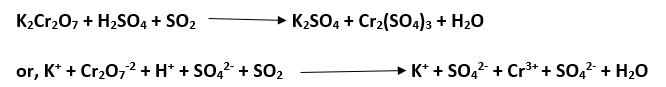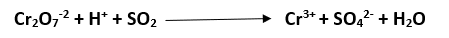# Ion-Electron Method↪ The method is based on the fact that electrons lost during oxidation half of any redox reaction are equal to the electrons gained during reduction half reaction but this method is limited to the redox reactions in which ions are involved.

﻿General Rules

1. If molecular equation is given, convert it into net ionic equation by cancelling spectator ions which are the ions present at both sides of all ionic equation and they are cancelled out while writing the net ionic equation.

↪ Writing ionic equation of a given molecular equation
(a) A molecular equation can't be transformed into ionic equation if the reaction medium is non-aqueous and the compounds present are all covalent.
(b) In aqueous medium, free elements, non-metallic hydrides and oxides remain in non ionic molecular state.
(c) Salts (except insoluble), acids and alkalis undergo ionization in aqueous solution.
(d) Spectator ions are cancelled out to get ionic equation.
Example: To write ionic equation of the given reaction.here, spectator ions are K+ and SO42- are cancel out
⇒ The net ionic equation is2. Indicate oxidation half and reduction half separately of ionic equation on the basis of concept of O.N. charge.

3. Balance oxidation half and reduction half separately at first in mass and then in their charge. Charge conservation is done by the loss of electrons in the equation of oxidation half and by the gain of electrons in the equation of reduction half. Here oxidant and reductant are those gaining and losing electrons respectively.

4. The balanced oxidation half and reduction half are then added together such that the total electrons must cancel out. Hence a complete balanced ionic equation can be obtained.

5. Finally, if required the balanced ionic equation can be converted to balanced molecular equation by adding those spectator ions at both sides of the equation which were cancelled out in course of writing ionic equation.